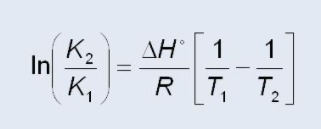# Problem: Nickel metal can be prepared by the reduction of nickel oxide:NiO(s)+CO(g) → CO2(g)+Ni(s)At 936, Kp = 4.54 x 103 and at 1125 K, Kp = 1.58 x103. Which statement is true?a. The reaction is endothermic.b. The activation energy decreases with increasing temperature.c. The reaction is exothermic.d. The activation energy increases with increasing temperature.

###### FREE Expert Solution

Recall that:

Exothermic reactions

lose energy, releases heat, temperature of the surroundings increases
▪ involves bond formation (for phase change: gas → liquid → solid)
ΔH is negative

Endothermic reactions

gain/requires energy, absorbs heat, temperature of the surroundings decreases
▪ involves breaking bonds (for phase change: solid → liquid → gas)
ΔH is positive

Van't Hoff Equation:96% (423 ratings)###### Problem Details

Nickel metal can be prepared by the reduction of nickel oxide:

NiO(s)+CO(g) → CO2(g)+Ni(s)

At 936, Kp = 4.54 x 103 and at 1125 K, Kp = 1.58 x103

Which statement is true?

a. The reaction is endothermic.

b. The activation energy decreases with increasing temperature.

c. The reaction is exothermic.

d. The activation energy increases with increasing temperature.